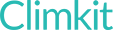# Configuring Modbus countersUpdated by Leah

### Standard meter model

The Modbus protocol allows the Gateway to read one or more specific data registers in a compatible meter.

Generally, electricity meters have an RS485-Modbus communication port, while heating and water meters communicate via Mbus.

Several models of Modbus meters are registered in the Climkit portal such as Eastron, Inepro, Schneider, ABB etc.

By selecting the correct model, the Gateway will automatically read the corresponding registers.

#### Modbus settings

By default, the Gateway reads meters and other Modbus devices with the following parameters:

• Baudrate: `9600`
• Parity: `None`
• Data Size: `8` bit
• Stop bits: `1` bit

This is also the default configuration for Eastron SDM630M meters.

Baudrate and parity can be changed in the meter directly through its interface.

Other models like the Inepro PRO380 and Polier MTR80LMOD have a different default parity configuration:

• Parity: `EVEN`

If you keep these default values, you must insert them in the additional parameters of the counter on the Climkit online portal:

We enter `E` for EVEN and `N` for NONE.

 `parity` `E`

In the case of using a counter whose model is not listed, you can manually configure the registers to read and specify what type of data it is.

Select the `generic_modbus` model and edit the additional parameters.

#### Parameters to set

Here is the list of parameters to define. Parameters marked with * are required.

` *reg <int>: register number to read from the meter. *unit <str>: unit of the read data (ex. 'L1_v') size <int>: data size (1, 2, 4, 8). Default is 1 for Int16 or Float16 factor <float>: multiples the data by the factor (ex. 0.001 to convert Wh to kWh) (float). Default is 1. pause <float>: sleep time after reading the data from a register. Default 0.3 for TCP meters and 0 for serial meters. function_code : Modbusbus Serial function code. Usually 3 or 4. Default 3. round <int> : Number of digits after the decimal point. Default 2. swap_words <bool or 1 or 0 >: reverse the registers order if size > 1.`
##### Unit

The `unit` parameter defines the type of data read.

For an electric meter the units are `en_im` (energy imported) and `en_ex` (energy exported).

For a heat meter: `heat_energy_kwh` , `cold_energy_kwh` , `flow_temp` , `return_temp` , `diff_temp` , `power_kw` , `vol_flow_m3_h` , `vol_m3`

For a water meter: `vol_m3`

##### Reg

The `reg` parameter defines the decimal position of the Modbus register to read. Refer to the documentation for the meter or device.

Here is an example for a thermal meter where the heat and cold energy indexes are recorded.

 `reg` `[{'unit': 'heat_energy_kwh', 'reg': 2544, 'size': 2, 'factor': 0.001}, {'unit': 'cold_energy_kwh', 'reg': 2546, 'size': 2, 'factor': 0.001}]`

Here is an example for an electric meter (type: `electricity` ) for which we only read the imported energy register in kWh.

 `reg` `[{'unit': 'en_im', 'reg': 1050, 'size': 2, 'swap_words': True}]`

### How Did We Do?

#### Configuring MBus Counters